HOME plateWON | World!OfNumbers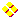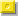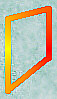Palindromic SquaresFactorizationRecordsSquares upto length 31Squares from length 32The SubsetsExtra Squarestrianglepentahexaheptaoctanonan(n+1)n(n+2)n(n+x)n^2+1n^2+xn^2–xn^2+(n+1)n^2+(n+x)

Introduction

Palindromic numbers are numbers which read the same from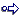left to right (forwards) as from the right to left (backwards)Here are a few random examples : 9, 9559, 4078704, 9876543210123456789

Square numbers are defined and calculated by this extraordinary intricate and excruciatingly complex formula.
So, this line is for experts onlyor ( base ) x ( base ) base 2
Here is a graphical representation of the first three square numbers to whet the beginner's appetite.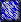square 1 square 4 square 9

 PLAIN TEXT SQUARES

Normal and Palindromic SquaresHere is the largest palindromic square with a non_palindromic non_core base
and was found by Feng Yuan from Washington State, USA on [ January 21, 2008 ].

 This basenumber 1.373.512.530.649.258.635.292.477.609has 28 digits yielding the following palindromic square record number with odd number of digits 1.886.536.671.850.530.641.991.373.196.913.731.991.460.350.581.766.356.881 having a staggering length of 55 digits.

An alternative way to get a 'structural' insight as how to imagine squares is to follow these links :

 SQUARES Acquaint yourself with SQUARES - by Richard Phillips From Eric Weisstein's Math Encyclopedia Someone gave me different numbers to find the square root of, including 14641.My answer was 121. Something special about this is that both numbers are readthe same left to right as right to left. Do you know any other numbers in whichboth the number and its square root are this way ?All palindromic square numbers can only start or end with digits 0, 1, 4, 5, 6 or 9.
Alas, my palindromes may not have leading 0's! So the zero option must not be investigated.
1 can only be followed by an even number : 10, 12, 14, 16 or 18
4 can only be followed by an even number : 40, 42, 44, 46 or 48
5 can only be followed by 2 : 52
6 can only be followed by an odd number : 61, 63, 65, 67 or 69
9 can only be followed by an even number : 90, 92, 94, 96 or 98There exist no palindromic squares of length  2, 4, 8, 10, 14, 18, 20, 24, 30, 38, 40.
(Sloane's A034822)

Palindromic Squares

Mike Keith made an original study of the palindromic squares.
He was able to classify and enumerate them in a logical manner.

• The trivial (Tr) Solutions - ex. 3 - the single digits.
• The Four Families
1. The Binary Root (B) Family - ex. 10010001110001001 - only with digits 0 and 1.
2. The Ternary Root (T) Family - ex. 100000020000001 - only with digits 0, 1, 2 and always odd.
3. The Even Root (E) Family - ex. 2000001000002 - only with digits (0,2) or (0,1,2) and always even.
4. The Asymmetric Root (A) Family - ex. 10109901101 or 10110n01101 - the pseudopalindromes.
• Sporadic Solutions - ex. 83163115486 - THE MOST INTERESTING ONES paradoxically!

Unlike Palindromic Triangulars where it is impossible to predict a next higher one, whether its basenumber is palindromic or not,
with the Palindromic Squares (and Cubes) we have an opposite situation. Finding a next higher number is very easy.
Start e.g. with the number 11. Then repeatedly add a zero between the two 'ones' and square them.
A pattern emerges that can go on forever.

BaseSquare
11121
10110201
10011002001
10001  100020001

There are other numbers with the same properties (e.g. 10101). The repunit numbers like '111' looks also a good candidate
for finding palindromic squares (not for cubes though) but the expansion doesn't go on forever. Nine 'ones' still produce
a palindrome when squared, but not ten 'ones'. It's a near miss as only the number 8 doesn't show up in the left part of the square.
This anomaly stays with longer repunits as well, so we must abandon this candidate.
If we take resort to higher basenumbers only then we can extend the pattern (for a while)!

BaseSquare
11
11121
11112321
11111234321
11111123454321
11111112345654321
11111111234567654321
11111111123456787654321
11111111112345678987654321
1111111111  1234567900987654321 = NOT palindromic in base 10 !

[ November 4, 2005 ]
Here is how Natalie Dickendasher (email) figures them out quickly in her head and impresses friends or boss :
The highest digit of the sequence will always be the quantity of 1's in the number your are squaring.
As an example lets start with my salary for next year (yah right): what is 111111 squared ?
EASY !! This number has a total of 6 digits. Therefore the highest number in the palindrome will be 6
so count to 6 and then back down. The answer is 12345654321.

Finding large palindromic squares is easy if you start with some palindromic basenumbers as explained above.
So, let's concentrate on the difficult ones namely those with a non palindromic basenumber. The first six are
(26) (264) (307) (836) (2285) and (2636) and can be found without too much trouble with a spreadsheet or calculator.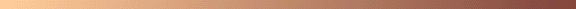Mike Bennett made an attempt in finding out what his chances are in discovering palindromic squares and palindromic cubes. Here is his mathematical analysis and the probability of findingthose interesting palindromic polygonal numbers.Sources Revealed

The second highest one (3069306930693) is copied from Martin Gardner's book "The Ambidextrous Universe"
see page 40.

Another source "Curious and Interesting Numbers" by David Wells, page 185, provided me the basenumber 798644.

While surfing the Internet I came across the work of Keith Devlin namely All the Math that's Fit to Print.
In chapters 17, 75 and 110 he printed some palindromic squares he got from other readers.
The highest one that originates from his publication is 6360832925898.

 Neil Sloane's "Integer Sequences" Encyclopedia can be consulted online : Neil Sloane's Integer Sequences The normal squares are categorised as follows : %N The squares: a(n) = n^2. under A000290 The palindromic squares are categorised in the following manner : %N Square is a palindrome. under A002778 %N Palindromic Squares. under A002779. Click here to view some of the author's [P. De Geest] entries to the table. Click here to view some entries to the table about palindromes.

In the mean time I was making a table of palindromic squares from scratch myself, hoping to find larger numbers
then the ones mentioned above. And in [ October 1996 ], I succeeded beginning from  !
(Un)fortunately soon afterwards this record was broken by submitters like Graham Lyons, Pete Leadbetter
and Mike Bennett who sent in numbers of even greater calibre ! Observe that the top three palindromic square
submitters were all British ! Was I detecting a new pattern here ?

William Rex Marshall calculated and posted [ February 2001 ] all the Palindromic Squares
of the sporadic type smaller than 1040. Three more palindromic squares sofar unknown to me
could be extracted and added to the list.
2281389294763414052 = 52047371142611077177011624117374025
4040997647536659812 = 163296619873968186681869378916692361
22822117694582308052 = 5208490560653668833388663560650948025
The second one is of even length 36, which surprised me as I thought that all these
would have been detected already... Somehow a few could escape detection.

Pete Leadbetter from England submitted the largest palindromic square with a non_palindromic non_core base
of 52 digits on [ May 20, 2001 ].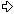648974001055156211773146822 = 4211672540455378958718869999688178598735540452761124
" Pete wrote that this square was found using an Athlon 1200 MHZ PC programmed
in Powerbasic about a third of the way into a 23 day search. The algorithm is basically
the same as the one used to find the previous 44 digit number. A faster machine
plus the more efficient compilation that Powerbasic affords allowed the 52 digit range
to be looked at within a 'not so mind boggling' time.
By the way, the find reported to you was the result of a 'burn in' for my new PC,
as was my previous 44 digit result! Also I'd recommend Powerbasic for number
crunching, it's seriously quick!

Pete remarks that of the set of EVEN length palindromic squares none of them
seems to end in digit 5. Is there a proof for this ? If the base ends in 5 then the
square must end in 25. Perhaps this reduces the freedom of one of the digits thereby
reducing the likelyhood of such a number. Or are there other number theory reasons ? "

Feng Yuan from Washington State, USA, sent in some new sporadic palindromic squares of 41 and 43 digits
[ March 30, 2002 ] [ March 31, 2002 ].
Website addressPalindromic Square Numbers
1) 1285011502365773736692 = 16512545612123429216861292432121654521561
2) 2293597822350854822252 = 52605909706925833964246933852960790950625
3) 2600888040530444070262 = 67646185993742928767376782924739958164676
4) 2633971384101768900862 = 69378052522669882013031028896622525087396
5) 11216211840338232315392 = 1258034080473435562012102655343740804308521
6) 20161925094267606635982 = 4065032235068578387346437838758605322305604
7) 22110079063202646738782 = 4888555961810720288016108820270181695558884
8) 30362334558547758656232 = 9218713598451835185192915815381548953178129
" The palindromic square number searching algorithm starts by assuming a
generic quadratic formula F(n) = An^2 + Bn + C, where A, B, and C are
non-negative integer numbers. Now the code tries to find palindrome number
when n is within a certain range 'from' to 'to'. It divides the range into
subranges such that for the first and last n within the range F(n) have the
same length and same first digit. For that range, it searches all the last
number of n within 0..9 such that the latest digit of F(n) matches the first
digit of F(n). Now we know when n is within that range, and when n is of the
form 10 * m + f, the first and last digit of F(n) are always the same (f).
After that the code substitute n = 10 * m + f into F(n), remove the highest
digit, divide by 10 to remove the last digit to get F(m). The routine is
called recursively to work on the new formula until the range is small
enough to work with using an iterative algorithm.

I'm using a 1.4Ghz Pentium 4 machine, running Windows XP. Program is
written in Microsoft Visual C++ 6.0. The time the algorithm needs to
complete search for n-digit palindrome squares is around O(10^(n/4)), that
is to say time increases 10 times for every 4 digits. Using the current
code, I estimate that it will take around 200 days to complete search all
the 52-digit numbers. I hope I can reduce that to something like 50 days. "

Feng Yuan from Washington State, USA, sent in four new sporadic palindromic squares of 45 digits
[ April 19, 2002 ]
Website addressPalindromic Square Numbers
1) 120933158072170133341212 = 146248287213084882816757618288480312782842641
2) 207061937167770337662682 = 428746458236696712033040330217696632854647824
3) 251456971046363576963942 = 632306082878117302583050385203711878280603236
4) 258865748030232616177262 = 670114755032518816023606320618815230557411076
" The complete search for 45-digit palindromic numbers can be completed
in around 30 hours. How is that done? I doubled the speed of my code and
got a 2.0 Ghz dual-CPU machine. "

Hidenori Takahashi [email] from Japan, sent in a new sporadic palindromic square of 48 digits
[ May 14, 2002 ]7229564563589573134345352 = 522666037791100950480675576084059001197730666225
" My name is Takahashi, and I live in Osaka. I am untrained and doesn't
know any English at all, so ask a Japanese to translate this message. ( ps. done so! )
Since I love mathematics, I was very interested when I saw these numbers.
In Japanese we call these palindromic squares 'kaibunheihousuu', and my
program is an improvement of Assembler code made 15 years ago.
Its efficiency is still bad, but because I investigate very thoroughly,
there are no unexpected slovenly errors. Palindromic squares will arise
66 days after the program starts when it is ready of course. I expect to
finish it the second half of July. "

Feng Yuan from Washington State, USA, sent in new sporadic palindromic squares of 47 & 49 digits
[ January 1-2, 2008 ]
1) 1365693915181949409770412 = 18651198699650016285556865558261005699689115681
2) 21075612070054235346324622 = 4441814241274157711392425242931177514721424181444
3) 21988344532547691788718722 = 4834872952820199705196890986915079910282592784384
" I found a new one last night using my 8-core machine.
Happy New Year. "

" The machine I'm using is Dell Precision PWS690, 2.33GHz. It's dual QuadCore, so 8 cores in total.
I'm trying to use all 8 cores at the same time to boost performance. The complete search for 47 digits
took 35,540 seconds (clock time), around 10 hours.
It's still 32-bit programs. 64-bit would be definitely faster, but I do not have it installed on my machine.
Searching 48 digits came back empty. Now I'm in the middle of searching for 49 digits. "

I found Feng Yuan's record palindromic square of 55 digits in the following archived blog
http://blogs.msdn.com/fyuan/archive/2008/01/31/sts-a-palindromic-word-i-will-remember.aspx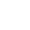Click here to displaythe full listing upto length 31.Click here to displaythe full listing from length 32 onwards.Pseudopalindromic Basenumbers

Careful observation of some numbers in the list lead me to the discovery of ever greater non-palindromic basenumbers whose squares are palindromic.
Specially the numbers with indexes , ,  and  attracted my attention because of the CORE number 091 in them.

 1109111
 110091011
 111091111
 11000910011
To the left there was the 'border' number 11 followed by 0, 1 and 2 zero's. To the right there was its reversal.
So without the core 091 we would have a palindromic basenumber but with 091 in the middle we always have
a non-palindromic basenumber ! The general layout becomes as follows :
11 + X_zero's + 091 + X_zero's + 11
No matter what value of X we always create a palindromic square.
The same is true if we substitute 11 with 111
111 + X_zero's + 091 + X_zero's + 111
I'm still investigating border values other then 11 or 111.
Borders like 1011 and 1101 also look promising...

A second promising CORE number exists. It is 09901 but it shows up only from .

 10109901101
 1010099010101
 1011099011101
 100110990111001
 101000990100101
 101010990110101
 10010109901101001
 10100009901000101
 10100109901100101
 10101009901010101
As for now, I can't discover a regularity in it... Can you ?
It looks that this CORE number likes to be surrounded by 101 and the number zero.
Yes, it gives us a method to produce infinite palindromic squares once more :
101 + X_zero's + 09901 + X_zero's + 101

I think a new third CORE number is emerging... It is 0999001
Watch out from

 100109990011001
 10010099900101001
 10011099900111001

Quite interesting. Let's put these three CORE numbers in a row :

091
09901
0999001
Extending the pattern to the next one gives this CORE number of 9 digits :
099990001
We also observed that the length of the CORE basenumbers increases with 2 and so their respective squarelengths with 4.
As the table already shows all the CORE numbers up to length 33, we must at least add 5 digits on both sides to start with
basenumbers of lengths 19. Let's try some combinations :
1000009999000100001 yields no palindromic square but
1000109999000110001  does yield a palindromic square !
Just by playing around with beautiful patterns we reveal more palindromic squares.
Wonderful !

David W. Wilson took up the thread where I left off.
[ February 16, 1998 ].

If we allow a digit "n" with value "–1", then 091 is equal to the palindrome 1n1,
while 09901 = 10n01, 0999001 = 100n001, etc. Thus

10109901101 = 10110n01101 = 
is a sort of 'pseudopalindrome'. When we square it, lo and behold
```
10110n01101
x 10110n01101
-----------
10110n01101
10110n01101..
10110n01101...
n0nn010nn0n.....
10110n01101.......
10110n01101........
10110n01101..........
---------------------
102210100272001012201
```
we obtain a palindrome because the sums of the individual rows happen to lie between 0 and 9 inclusive.
Here's a palindromic square family :
111n1112 = 11091112 = 1230127210321 = 
101010n0101012 = 10100990101012 = 1020300010207020100030201 = 
100100100n0010010012 = 10010009990010010012 = 1002003000001002007002001000003002001 = 
etc.
I think you get the picture. The family is infinite, as is the family
1111n11112 = 
10101010n010101012 = 
etc.
In fact, any pseudopalindrome with a palindromic square can be interspersed with equal numbers of zeroes
to generate another pseudopalindrome with a palindromic square.What are the conditions for creating a pseudopalindrome with a palindromic square ? Open this window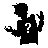and read David W. Wilson's answer.Mike Keith made an original study of the palindromic squares.
He was able to classify and enumerate them in a logical manner,
and went even further as he tried also to create a general formula for
calculating the number of certain classes of palindromic squares, except for the 'sporadic' solutions.

• The trivial (Tr) Solutions - ex. 3 - the single digits.
• The Four Families
1. The Binary Root (B) Family - ex. 10010001110001001 - only with digits 0 and 1.
2. The Ternary Root (T) Family - ex. 100000020000001 - only with digits 0, 1, 2 and always odd.
3. The Even Root (E) Family - ex. 2000001000002 - only with digits (0,2) or (0,1,2) and always even.
4. The Asymmetric Root (A) Family - ex. 10109901101 or 10110n01101 - the pseudopalindromes.
• Sporadic Solutions - ex. 83163115486 - THE MOST INTERESTING ONES paradoxically!
Mike had most trouble with the Asymmetric Root Family but thanks to
Dave Wilson's above "lemma" he made significant progress.

More Patterns

and that is finalized alternatively with a chopped off and rounded version of it namely a 3 or 307...

 3
 307
 3069 3
 3069 307
 3069 3069 3
 3069 3069 307
 3069 3069 3069 3
Each time the length increases with 2
and the length of the resulting squares increases with 4.
So, logically the next one should be 306930693069307 but alas... the square is NOT palindromic !
Oh well, it can not work every time ! On [ January 5, 1997 ] this near miss popped up on my
computerscreen : 306950094269977057. The system surely knows how to comfort me...

3069 occurs as displacements to powers of ten so that this number forms the nearest (probable) prime to those axes :

 101001 – 3069 is the largest prime of 1001 digits 105068 – 3069 is the largest prime of 5068 digits 107565 – 3069 is the largest prime of 7565 digits 1019217 – 3069 is the largest prime of 19217 digits 1028898 – 3069 is the largest prime of 28898 digits 101555 + 3069 is the smallest prime of 1556 digits 102399 + 3069 is the smallest prime of 2400 digits 105016 + 3069 is the smallest prime of 5017 digits 105063 + 3069 is the smallest prime of 5064 digits 109896 + 3069 is the smallest prime of 9897 digits

NEVER ODD OR EVENRead title backwards please !

It looks as though the decent sufficiently-random nonpatternlike unpredictable nonpalindromic basenumbers become very rare.
In the table (see Full Listing or the Subsets) I highlighted them using a lightblue background in the side-cells.
And even then a further distinction can be made. Consider the even and odd length values for a minute.
Palindromic squares with an even length are just a plain minority. Until now I can only list sixteen of them.
These are highlighted in the table by means of a aquamarine background color in the side-cells.
SeeSporadic Palindromic Squares of EVEN length

I 'almost' solved a very ancient problem namely : squaring the circle (pi = 3,1415....)
MORELAND PI welcomes PALINDROMES

    314155324482867

This basenumber starts with the the number 2201 which is (to me) the only known nonpalindromic basenumber
of a palindromic cube ! The cube of 2201 equalling 10662526601.

    2201019508986478

Here's another attractive but finite expansion starts with the number 1 whereby 01 is repeatedly added.
The following series arises :
BaseSquare
11
10110201
10101102030201
10101011020304030201
10101010110203040504030201
10101010101102030405060504030201
10101010101011020304050607060504030201
10101010101010110203040506070807060504030201
10101010101010101102030405060708090807060504030201 (° see conjecture below)
1010101010101010101  10203040506070809100908070605004030201 = QUITE UNpalindromic !

CONFIRMATION
10101010101010101 is indeed the smallest integer that generates
a palindromic square 102030405060708090807060504030201
that is also pandigital (contains all the ten digits).

Here is a good place to talk about a famous conjecture that nobody
could prove or disprove for more than 80 years.
I must thank Mike Keith for letting me know this fact through an email message.
Mike has heard that this conjecture originates from
L. E. Dickson's famous "History of the Theory of Numbers".

History :
My table lists exhaustively all palindromic squares upto lengths 31.
I also listed all the palindromic squares of length 32.
Palindromic squares of 'even' nature seem to be very popular among the record
breaking submitters. They told me there are no gaps for the 'even' length 32 !
So, in fact I had to finish checking only length 33...
Of which I checked all squares beginning with 1, 5 and 9 !
( and 50 % of those beginning with digits 4 and 6 ).
I collected a total of 151 palindromic squares beginning with digit 1.
(Un)lucky for me Dickson's number 102030405060708090807060504030201
( 101010101010101012 ) is the 86th one. Anyway after checking these 86 squares
I could proudly announce that the conjecture was finally proven [ May 3, 1998 ].
The second smallest number (?) that answers to these rules may be this one (also of length 33) :
118431915157648212 = 140261185279083838380972581162041.Sloane's A001110 gives the first numbers that are both Square and Triangular.
1, 36, 1225, 41616, 1413721, 48024900, ...
Consult also Eric Weisstein's page Square Triangular NumberSloane's A036353 gives the first numbers that are both Square and Pentagonal.
1, 9801, 94109401, 903638458801, 8676736387298001, ...
Consult also Eric Weisstein's page Square Pentagonal Number.Sloane's A036354 gives the first numbers that are both Square and Heptagonal.
1, 81, 5929, 2307361, 168662169, 12328771225, 4797839017609, ...
Consult also Eric Weisstein's page Square Heptagonal Number.Sloane's A036428 gives the first numbers that are both Square and Octagonal.
1, 225, 43681, 8473921, 1643897025, ...
Consult also Eric Weisstein's page Square Octagonal Number.Sloane's A036411 gives the first numbers that are both Square and Nonagonal.
1, 9, 1089, 8281, 978121, 7436529, ...
Consult also Eric Weisstein's page Square Nonagonal Number.
```

```Click here to displaythe subsets of palindromic squares.Contributions

Feng Yuan (email) from Washington State, USA, sent a record sporadic palindromic square 53 of digits [ January 16, 2008 ].

Feng Yuan (email) from Washington State, USA, sent a palindromic square with even numbers 50 of digits [ January 8, 2008 ].

Feng Yuan (email) from Washington State, USA, sent record palindromic squares with odd numbers 47 & 49 of digits [ January 1-2, 2008 ].
Website addressPalindromic Square Numbers
Go to local paragraph

Hidenori Takahashi (email) from Japan, sent in a new sporadic palindromic square of 48 digits [ May 14, 2002 ]
Go to local paragraph

Feng Yuan (email) from Washington State, USA, sent four new sporadic palindromic squares of 45 digits [ April 19, 2002 ].
Website addressPalindromic Square Numbers
Go to local paragraph

Feng Yuan (email) from Washington State, USA, sent five new sporadic palindromic squares of 41 and 43 digits [ March 30, 2002 ].
Website addressPalindromic Square Numbers
Go to local paragraph

Many thanks go to Pete Leadbetter who submitted this number of 52 digits [ May 20, 2001 ].
Base    64897400105515621177314682 [length 26]
Square 4211672540455378958718869999688178598735540452761124 [length 52]

Pete Leadbetter submitted also this number of 44 digits [ June 27, 1997 ].
Base    3823177109095314778621 [length 22]
Square 14616683207510408440555504480401570238661641 [length 44]

Pete Leadbetter informs me that he checked for 40 digit palindromes and found none.
He's convinced that there aren't any 38's since his 'old' program searched that far.
There are no more 44 digit squares that are palindromic other than the above one.
In his letter he says also that for the case of 42 digits it will take nearly as long (12 days on his PC !)
and leave the search for someone else with enough free computer timecycles.

Many thanks go to Graham Lyons (email) who submitted the following number of 36 digits [ April 29, 1997 ].
Base    795559265009384106 [length 18]
Square 632914544142271449944172241445419236 [length 36]

The first even palindromic square of length 32 was also submitted by Graham Lyons
Base    6819209882215742 [length 16]
Square 46501623417708833880771432610564 [length 32]

Congratulations also to Mike Bennett (email) who submitted the following even digit palindromic squares of 36, 42 and 48 digits
as recently as [ November 29, 1997 ].
Base    633856150760638652 [length 18]
Square 401773619857093475574390758916377104 [length 36]
and the following a few days later [ December 5, 1997 ].
Base    637323988797048057098 [length 21]
Square 406181866696179837389983738971696668181604 [length 42]
and finally this actual record number sent [ January 13, 1998 ].
Base    831775153121251039203514 [length 24]
Square 691849905349880612384525525483216088943509948196 [length 48]
Mike found these palindromes using an algorithm in C++ that runs on an Acorn RiscPC with a StrongARM processor.
He also confirms that there are no 38 or 40 digit solutions.
Regarding the time to test for all 46 and 48 digit palindromes Mike wrote me that he estimates this needs about 150 days.
He tests for numbers of the form 4n and 4n-2 in parallel. The 48 digit record palindrome was a fluke that turned up in less than 1 day !

Alain Bex (email) gave also some palindromic squares in base 12.
Link to Palindromic Numbers other than Base 10

David W. Wilson's (email) contribution regarding the 'Pseudopalindromes with a palindromic square'
is very remarkable and therefore much appreciated.

Mike Keith (email) (website) published an article about palindromic squares in the "Journal of Recreational Mathematics",
Volume 22, Number 2 - 1990, pp. 124-132, titled "Classification and enumeration of palindromic squares",
and was followed by an article by Charles Ashbacher, pp. 133-135, "More on palindromic squares".

```

```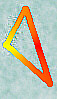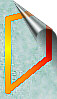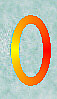[TOP OF PAGE]

Patrick De Geest - Belgium- Short Bio - Some Pictures